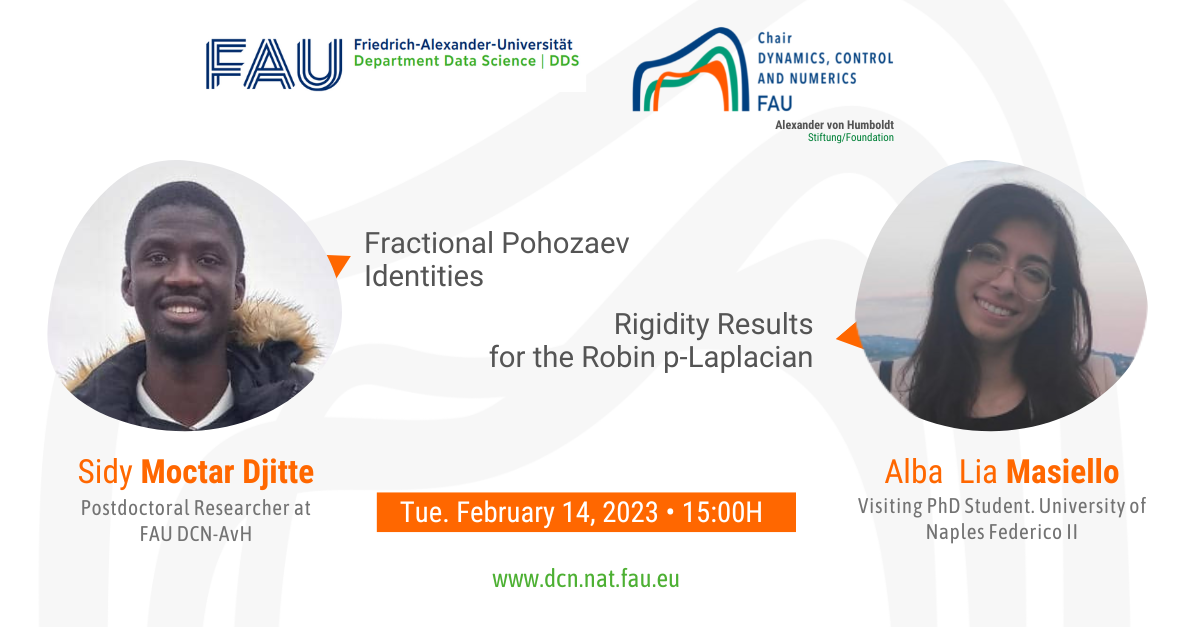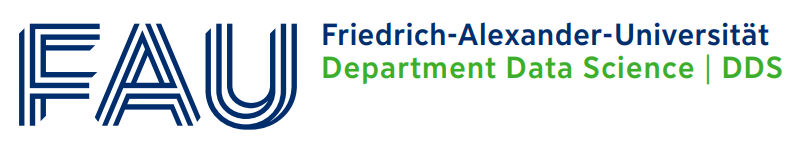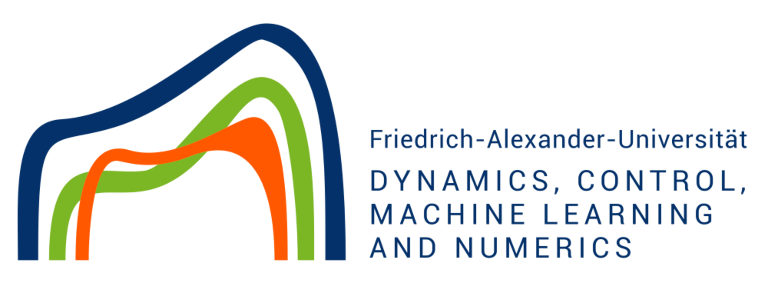# Mini-workshop: Variational Methods, Functional Inequalities, and Shape Optimization

Next Tuesday, February 14, 2023:

Event: FAU DCN-AvH Mini-workshop: Variational Methods, Functional Inequalities, and Shape Optimization
Organized by: FAU DCN-AvH, Chair for Dynamics, Control and Numerics – Alexander von Humboldt Professorship at FAU, Friedrich-Alexander-Universität Erlangen-Nürnberg (Germany)

15:00H
Title: Fractional Pohozaev Identities
Speaker: Dr. Sidy Moctar Djitte
Affiliation: Postdoctoral researcher at FAU DCN-AvH
Abstract. Pohozaev identities has become an essential tool in analysis of PDEs. Among other things, it guarantees uniqueness of solutions of supercritical semilinear Dirichlet problems. It is also an important tool in control theory of evolution equations. In this talk, we prove a generalised fractional Pohozaev identity and discuss its application. Specifically, we shall consider applications to nonexistence of solutions in the case of supercritical semilinear Dirichlet problems and regarding a Hadamard formula for the derivative of Dirichlet eigenvalues of the fractional Laplacian with respect to domain deformations. We also applied the identity to give an alternative proof of some classical improved Hardy type inequalities. We end the talk by stating some open problems and future research directions.

The talk will be based on the following works
• A generalised Pohozaev identity and applications (S. M. D, M. M. Fall, and Tobias Weth), to appear in Advances in Calc of Var.
• Fractional Hardy-Rellich inequalities via a Pohozaev identity (S.M. Djitte, and Nicola De Nitti), submitted.

15:35H
Title: Rigidity Results for the Robin p-Laplacian
Speaker: Alba Lia Masiello
Affiliation: Visiting PhD Student from University of Naples Federico II
Abstract.Let \Omega\subset\mathbb{R}^n, n\geq 2, be a bounded, open and Lipschitz set and let f be a positive function. We consider the following problem

\begin{cases} -\Delta_p u:= -\div(\vert{\nabla u}^{p-2} \nabla u)=f & \text{ in } \Omega \\[1ex] \vert{\nabla u}^{p-2} \displaystyle{\frac{\partial u}{\partial \nu}} + \beta \vert{u}^{p-2}u =0 & \text{ on } \partial \Omega, \end{cases} \quad \, \;(1)

where \nu is the unit exterior normal to \partial\Omega and \beta>0, and its symmetrized version

\begin{cases} -\Delta_p v=f^\sharp & \text{ in } \Omega^\sharp \\[1ex] \vert{\nabla v}^{p-2} \displaystyle{\frac{\partial v}{\partial \nu}} + \beta \vert{v}^{p-2}v =0 & \text{ on } \partial \Omega^\sharp, \end{cases} \quad \, \;(2)

where \Omega^\sharp is the ball centered at the origin with the same measure of \Omega.

In [1,2] the authors prove a comparison á la Talenti between the solutions to equations (1) and (2).
In particular, they prove

\parallel{u}_{L^{pk,p}(\Omega)} \, \leq \parallel{v}_{L^{pk,p}(\Omega^\sharp)}, \quad \, \; \forall \, 0 \lt k \leq \frac{n(p-1)}{(n-2)p +n}, \quad \, \;(3)

and in the case f\equiv 1, they prove

\parallel{u}_{L^{pk,p}(\Omega)} \, \leq \parallel{v}_{L^{pk,p}(\Omega^\sharp)}, \quad \, \; \forall \, 0 \lt k \leq \frac{n(p-1)}{(n-2)p +n}, \quad \forall p>1, \quad \, \;(4)

where \parallel{\cdot}\parallel_{k,q} is the Lorentz norm of a measurable function.

In this seminar, we are interested in characterizing the equality cases in (3) and (4), proving that these estimates are rigid, i.e. the equality case can occur only in the symmetric setting.
This is a joint work with Gloria Paoli.

References
 A. Alvino, C. Nitsch, and C. Trombetti. A Talenti comparison result for solutions to elliptic problems with Robin boundary conditions. to appear on Comm. Pure Appl. Math.
 V. Amato, A. Gentile, and A. L. Masiello. Comparison results for solutions to p-Laplace equations with Robin boundary conditions. Ann. Mat. Pura Appl. (4), 201(3):1189–1212, 2022.

##### WHERE?

On-site / Online

On-site:
Room Übung 4 | 01.253-128. 1st. floor
Friedrich-Alexander-Universität Erlangen-Nürnberg
Cauerstraße 11, 91058 Erlangen
GPS-Koord. Raum: 49.573639N, 11.030503E

Online:
Meeting ID: 614 4658 159 | PIN code: 914397

### Date

Tue. Feb 14, 2023
Expired!

### Time

15:00 - 16:15### Location### Organizer

###### Website
http://www.dcn.nat.fau.eu

### Speakers

•Alba Lia Masiello
Visiting PhD Student from University of Naples Federico II
•Sidy Moctar Djitte
Postdoc at FAU DCN-AvH